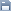Leetcode
2020.04.14 14:20

조회 수 96 추천 수 0 댓글 0
?

#### 단축키

Prev이전 문서

Next다음 문서

크게 작게 위로 아래로 댓글로 가기 인쇄
?

#### 단축키

Prev이전 문서

Next다음 문서

크게 작게 위로 아래로 댓글로 가기 인쇄

You are given two non-empty linked lists representing two non-negative integers. The digits are stored in reverse order and each of their nodes contain a single digit. Add the two numbers and return it as a linked list.

You may assume the two numbers do not contain any leading zero, except the number 0 itself.

Example:

```Input: (2 -> 4 -> 3) + (5 -> 6 -> 4)
Output: 7 -> 0 -> 8
Explanation: 342 + 465 = 807.```

```/**
* public class ListNode {
*     int val;
*     ListNode next;
*     ListNode(int x) { val = x; }
* }
*/
class Solution {
public ListNode addTwoNumbers(ListNode l1, ListNode l2) {
ListNode fake = new ListNode(0);
ListNode p = fake;

ListNode p1 = l1;
ListNode p2 = l2;

int carry = 0;

while(p1 != null || p2 != null){
int sum = carry;
if(p1!=null){
sum += p1.val;
p1 = p1.next;
}

if(p2!=null){
sum += p2.val;
p2 = p2.next;
}

if(sum>9){
carry=1;
sum = sum-10;
}else{
carry = 0;
}

ListNode l = new ListNode(sum);
p.next = l;
p = p.next;
}

//don't forget check the carry value at the end
if(carry > 0){
ListNode l = new ListNode(carry);
p.next = l;
}
return fake.next;
}
}```

?

 제목+내용제목내용댓글이름닉네임아이디태그
List of Articles
번호 분류 제목 글쓴이 날짜 조회 수
17 Leetcode 287. Find the Duplicate Number 2020.04.16 126
16 Leetcode 253. Meeting Rooms II 2020.04.15 104
15 Leetcode 731. My Calendar II 2020.04.15 100
14 Leetcode 729. My Calendar I 2020.04.15 104
13 Leetcode 692. Top K Frequent Words 2020.04.15 93
12 Leetcode 973. K Closest Points to Origin 2020.04.15 107
11 Leetcode 75. Sort Colors 2020.04.14 101
» Leetcode 2. Add Two Numbers 2020.04.14 96
9 Leetcode 23. Merge k Sorted Lists 2020.04.14 104
8 Leetcode 347. Top K Frequent Elements 2020.04.14 102
7 Leetcode 994. Rotting Oranges2020.04.14 115
6 Leetcode 3. Longest Substring Without Repeating Characters 2020.04.09 113
5 Leetcode 62. Unique Paths2020.04.09 105
4 Leetcode 946. Validate Stack Sequences 2020.04.08 107
3 Leetcode 114. Flatten Binary Tree to Linked List 2020.04.06 117
2 Leetcode 430. Flatten a Multilevel Doubly Linked List2020.04.06 104
1 Leetcode 1055. Shortest Way to Form String 2020.04.06 125
Board Pagination Prev 1 2 3 4 Next
/ 4